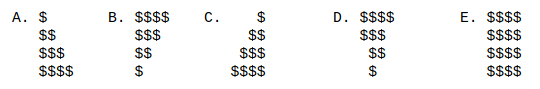# Subgoals

## Section5.13Loops-WE6-P1

### Subsection5.13.1Subgoals for Evaluating a Loop

1. Diagram which statements go together.
2. Define and initialize variables
1. Determine the start condition.
2. Determine the update condition.
3. Determine the termination condition.
4. Determine body that is repeated.
3. Trace the loop.
1. For every iteration of the loop, write down the values.

### Subsection5.13.2Loops-WE6-P1

#### ExercisesExercises

##### 1.
Q15: What is the output of the following loop?
for (int m = 0; m < 4; m++)
{
for (int n = 5; n >= 0; n--)
{
System.out.print("#");
}
System.out.println();
}

• 5 lines of ###
• 3 lines of #####
• 4 lines of ######
• 5 lines of ####
• Compilation error
##### 2.
Q16: What is the output of the following loop?
for (int m = 0; m < 4; m++)
{
for (int n = 0; n <= m; n++)
{
System.out.print("$"); } System.out.println(); }Figure 5.13.1. • See diagram for answer A • See diagram for answer B • See diagram for answer C • See diagram for answer D • See diagram for answer E ##### 3. Q17: What is the output of the following loop? for (int m = 1; m < 5; m++) { for (int n = 1; n <= m; n++) { System.out.print(" "); } System.out.println("$");
}